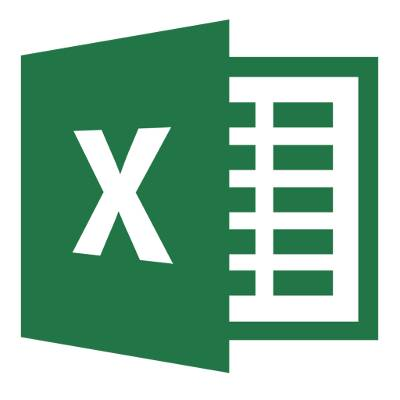# Understanding Basic Formulas in Microsoft Excel 2013## Understanding Basic Formulas in Microsoft Excel 2013It can be difficult to keep track of your budget and expenses, especially when prices and needs are always changing. But perhaps the biggest annoyance is the intense paper trail that you leave behind when building your budget. By taking advantage of Microsoft Excel’s formulas, you can easily keep track of your budget and alter it as prices change and demand increases.

Mathematical Orders
Excel operates similarly to a calculator, and it has several mathematical functions that it can run:

Subtraction: –
Multiplication: *
Division: /
Exponents: ^

In order to initiate a calculation sequence, begin the formula with an equals sign (=). The cell which holds the formula will equal the result of the calculation. For example: =5+3 would create the number 8 in the selected cell.

Cell References
While you can enter Excel formulas into cells manually, you can also use cell addresses to enter a formula into a spreadsheet. Cell addresses generally use a combination of letters and numbers to determine the location of the cell on the chart. If you take a look at the columns and rows, you’ll notice they are marked with letters and numbers, and these determine the address of the cell. By using a combination of cell addresses when entering formulas, you can guarantee accurate results for calculations. This is imperative when working with a strict budget. For example: =A3*A5 or =B2-A1

These cell addresses are used to represent the value of the entered cell. You can also use a combination of cell addresses and set values, like so: =C6/2 or D1*4 or B6^2

Brewing Formulas
It has never been easier to build a basic budget spreadsheet in Microsoft Excel 2013.

1. Select a cell. This is the beginning point for the formula. We’ll use B3 as an example.
2. Enter a formula into the formula bar at the top of the spreadsheet. Notice that the data entered into the formula bar will also appear in the selected cell.
3. Type the cell address of a cell that is to be used in the formula. Let’s say that cell B1 has the information for a budget surplus from December 2013, while B2 has January 2014’s budget. Those numbers will be added together. All you need to do is type B1 into the formula bar, and the cell will gain a blue border. This indicates that the cell will be used in the formula.
4. Type the address of another cell with a mathematical operator. For example, we want to add B1 and B2. The second reference cell will gain a red border.
5. Press Enter. The formula will be calculated and place the value in the selected cell. If the formula is too big to display the selected cell, it may appear as pound signs (or, for those who are unfamiliar with this enigma, it can also be called a #hashtag). To fix this, increase the width of the column.

Easily Modify Values and Formulas
Perhaps you have decided to increase the marketing budget for the next year, and you need the spreadsheet to represent this change. It’s as easy as changing the value in one cell. Excel will automatically recalculate the value of the formula after you edit one of the cells. It’s important to make sure that the calculation is correct, though, since Excel will not inform you if the recalculated value is invalid or not.

Optimizing your use of spreadsheets can contribute to more productivity in the long run for both your business and your employees. You can plan for the future and get ahead on your company’s asset management. For more tips and tricks, contact Resolve I.T. at (978) 993-8038. You’ll be surprised by how much time you can save by taking advantage of the latest Microsoft Office applications.

• SHARE
• TWEET